The Learning Point‎ > ‎Mathematics‎ > ‎

### Trigonometry 1a - Intro to Trigonometric Ratios, Identities and Formulas

We will cover:

Angles in Degrees and Radians, Introduction to Trigonometric Ratios, Domains and ranges, Basic Identities, Compound Angle Formulas, Double and Triple Angle Formulas

## Trigonometry Tutorials: At a glanceTrigonometry 1a ( Introduction to Trigonometry - Definitions, Formulas ) Trigonometry 1b ( Problems based on Trigonometric ratios ) Trigonometry 2a ( Basic concepts related to Heights and Distances ) Trigonometry 2b ( Problems on Heights, Distances and applications Trigonometry 3a ( Introducing Inverse Trigonometric Ratios) Trigonometry 3b (Problems related to inverse trigonometric ratios Trigonometry 4 ( A tutorial on solving trigonometric equations

--------xxx-----------

Trigonometry -1: Basic Trigonometric Ratios and Identities

### Target Audience: High School Students, College Freshmen and Sophomores, Class 11/12 Students in India preparing for ISC/CBSE and Entrance Examinations like the IIT-JEE, Anyone else who needs this Tutorial as a reference!

Important points to remember:

• ### Angle

A measure of rotation of a given ray about its initial point. The original ray is called the initial side and the final position of the ray after rotation is called the terminal side of the angle. The point of rotation is called the vertex. If the direction of rotation is anticlockwise, the angle is said to be positive and if the direction of rotation is clockwise, then the angle is negative.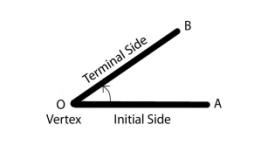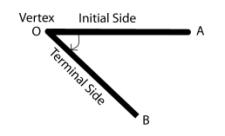Figure 1(i) - Positive Angle            Figure 1(ii) Negative Angle
• Degree measure: If a rotation from the initial side to terminal side is (1360)th of a revolution, the angle is said to have a measure of one degree, written as 1°. A degree is divided into 60 minutes, and a minute is divided into 60 seconds. One sixtieth of a degree is called a minute, written as 1′, and one sixtieth of a minute is called a second, written as 1″.Thus, 1° = 60′,1′= 60″
• Radian measure: There is another unit for measurement of an angle, called the radian measure. Angle subtended at the centre by an arc of length 1 unit in a unit circle (circle of radius 1 unit) is said to have a measure of 1 radian.
• If in a circle of radius r, an arc of length l subtends an angle θ radian at the centre, we have l=θ/r.

 Degree 30o 45o 60o 90o 180o 270o 360o Radian π/6 π/4 π/3 π/2 π 3π/2 2π

### Trigonometric ratios

A right angled triangle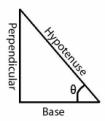• sinθ= perpendicular/hypotenuse

• cosθ= base/hypotenuse

• tanθ= perpendicular/base , θ ≠  (π/2)(2n +1) where n is any integer

• cotθ=1/tanθ , θ ≠nπ, where n is any integer

• secθ=1/cosθ , θ ≠ (π/2)(2n + 1) where n is any integer

• cosecθ=1/sinθ , θ ≠ nπ where n is any integer.

### Signs of trigonometric functions:

 Quadrant I II III IV sin + + - - cos + - - + tan + - + - cot + - + - sec + - - + cosec + + - -

### Domains and ranges of trigonometric functions:

• Domain of y = sin x and y = cos x is the set of all real numbers and range is the interval [–1, 1], i.e., – 1 ≤ y ≤ 1.
• The domain of y = cosec x is the set {x: x ϵ R and x ≠ n π, n ϵ Z} and range is the set {y: y ϵ R, y ≥ 1 or y ≤– 1}.
• The domain of y = sec x is the set {x: x ϵ R and x ≠ π2(2n + 1), n ϵ Z} and range is the set{y: y ϵ R, y≤ -1or y ≥ 1}.
• The domain of y = tan x is the set {x: x ϵ R and x ≠  π2(2n +1), n ϵ Z} and range is the set of all real numbers.
• The domain of y = cot x is the set {x: x ϵ R and x ≠n π , n ϵ Z} and the range is the set of all real numbers.

### Monotonicity of trigonometric functions:

 Quadrant I II III IV sin Increases from 0 to 1 Decreases from 1 to 0 Decreases from 0 to -1 Increases from -1 to 0 cos Decreases from 1 to 0 Decreases from 0 to -1 Increases from -1 to 0 Increases from 0 to 1 tan Increases from 0 to ∞ Increases from  -∞ to 0 Increases from 0 to ∞ Increases from  -∞ to 0 cot Decreases from  ∞ to 0 Decreases from 0 to -∞ decreases from  ∞ to 0 Decreases from 0 to -∞ sec Increases from 1 to ∞ Increases from -∞ to -1 Decreases from -1 to -∞ Decreases from ∞ to 1 cosec Decreases from  ∞ to 1 Increases from 1 to ∞ Increases from -∞ to -1 Decreases from -1 to -∞

### Plots of trigonometric functions:

#### y=sinx

•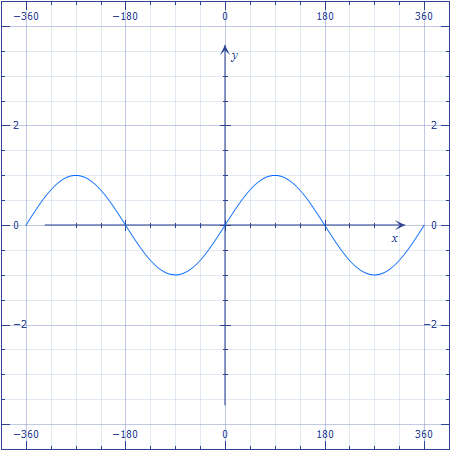#### y=cosx

•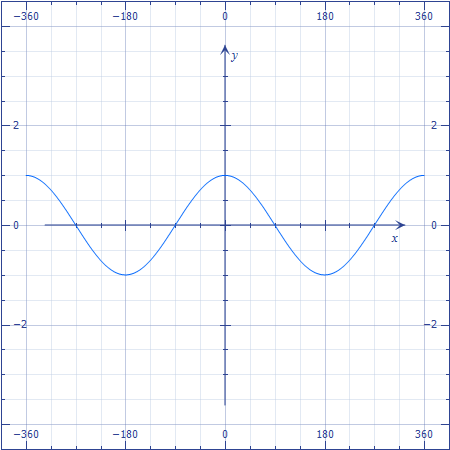#### y=tanx

•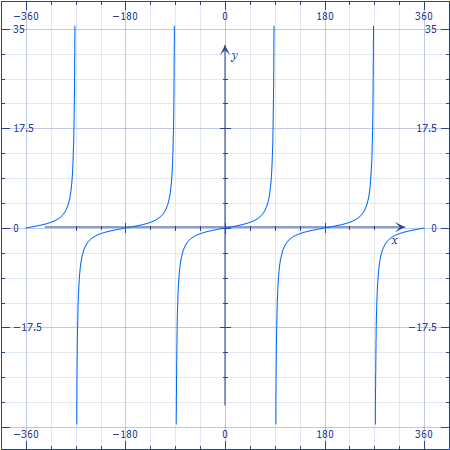### Basic Identities:

• sin2 x + cos2 x = 1
• 1 + tan2 x = sec2 x
• 1 + cot2 x = cosec2 x
• cos (– x) = cos x
• sin (– x) = – sin x

### Trigonometric Functions of Sum and Difference of Two Angles

• cos (x + y) = cos x cos y – sin x sin y
• cos (x – y) = cos x cos y + sin x sin y
• cos (π2  – x) = sin x & sin (π2  – x) = cos x
• sin (x + y) = sin x cos y + cos x sin y
• sin (x – y) = sin x cos y – cos x sin y
• cos (π2  + x) = – sin x  & sin (π2  + x) = cos x
• cos (π – x) = – cos x  & sin (π – x) = sin x
• cos (π + x) = – cos x  & sin (π + x) = – sin x
• cos (2π – x) = cos x  & sin (2π – x) = – sin x
• tan (x + y) = tanx+tany1-tanxtany
• tan (x – y) =tanx-tany1+tanxtany
• cot ( x + y) =cotxcoty-1coty+cotx
• cot (x – y)= cotxcoty+1coty-cotx
• ### Double Angle Formulae

• cos 2x = cos2x – sin2 x = 2 cos2 x – 1 = 1 – 2 sin2 x = 1-tan2x1+tan2x
• sin 2x = 2 sinx cos x =2tanx1+tan2x
• tan 2x =2tanx1-tan2x

• ### Triple Angle Formulae

• sin 3x = 3 sin x – 4 sin3 x
• cos 3x= 4 cos3 x – 3 cos x
• tan 3x =3tanx-tan3x1-3tan2x

### Sum to Product Formulae

• cos x + cos y =2cos(x + y2) cos(x- y2)
• cos x – cos y = –2sin(x + y2) sin(x- y2)
• sin x + sin y = 2sin(x + y2) cos(x- y2)
• sin x – sin y =2cos(x + y2) sin(x- y2)

### Product to Sum Formulae

• 2 cos x cos y = cos (x + y) + cos (x – y)
• –2 sin x sin y = cos (x + y) – cos (x – y)
• 2 sin x cos y = sin (x + y) + sin (x – y)
• 2 cos x sin y = sin (x + y) – sin (x – y).

### General solutions of trigonometric equations

• For any real numbers x and y, sin x = sin y implies x = nπ + (–1)n y, where n ϵ Z
• For any real numbers x and y, cos x = cos y, implies x = 2nπ ± y, where nϵZ
• if x and y are not odd multiple of  π2, then tan x = tan y implies x = nπ + y, where n ϵ Z

### Principle solutions

Those general solutions which lie between 0 to 2π i.e. 0≤x≤2 π.

#### In case you'd like to take a look at other Trigonometry tutorials :

 Trigonometry 1a ( Introduction to Trigonometry - Definitions, Formulas ) Introducing trigonometric ratios, plots of trigonometric functions, compound angle formulas. Domains and ranges of trigonometric functions, monotonicity of trigonometric functions quadrant wise. Formulas for double and triple angle ratios. Trigonometry 1b ( Tutorial with solved problems based on Trigonometric ratios ) Problems based on the concepts introduced above. Trigonometry 2a ( Basic concepts related to Heights and Distances ) Applying trigonometry to problems involving heights and distances. Angles of elevation and depression. Sine and Cosine rule, half angle formulas. Circumradius, inradius and escribed radius. Circumcentre, incentre, centroid and median of a triangle. Trigonometry 2b ( Tutorial with solved problems related to Heights and Distances and other applications of Trigonometry ) - Problems based on the concepts introduced above. Trigonometry 3a ( Introducing Inverse Trigonometric Ratios)Inverse trigonometric ratios - their domains, ranges and plots. Trigonometry 3b ( Tutorial with solved problems related to inverse trigonometric ratios )- Problems related to inverse trigonometric ratios. Trigonometry 4 ( A tutorial on solving trigonometric equations )- Solving trigonometric equations. Methods and transformations frequently used in solving such equations.